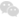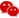关注我们# 数学自学考试函数公式

数学是自学考试中常考的科目之一，以下就是深圳市罗湖区人才培训中心关于数学自学考试的函数公式，方便同学们学习使用。

数学自学考试函数公式如下：

 序 章节 公式 公式 1 基础公式 (a+b)(a-b)=a2-b2 (a+b) (a+b)= (a+b)2 (a+b)2=a2+b2+2ab (a-b)2=a2+b2-2ab− ± √ 2 − 4= 2 b2 − 4ac = ∆ 2 集合 自然数集：N 正整数集：N+ 属于、不属于 ∈ ∉ 包含于、不包含于 ⊆ ⊈ 整数集：Z 实数集：R 交集、并集 ∩ ∪ 有理数集：Q 空集：∅ 全集、补集 I、Cu 3 函数 奇偶性：f(-x)=-f(x)为奇函数 f(-x)=f(x)为偶函数 以 Y 轴对称为偶函数 以原点对称为奇函数 单调性：x1f(x2) 则f(x)为减函数 奇+奇=奇 偶+偶=偶奇+偶=非奇偶 奇 x 奇=偶 偶 x  偶=偶 奇 x 偶=奇 4 图象 正比例函数 y=kx (常数k≠0) 一次函数 y=kx+b (常数k≠0) k 反比例函数 y= x (常k≠0 x≠0) 指数函数与对数函数 y=ax (a>0a≠1) y=logax (a>0a≠1) y=ax2+bx+c   b顶点坐标：(−     2a , 4ac−b                   4a 2 ) 图象 y=ax2+bx+c b 最值： 4ac−b2 5 二次函数2a 4a 求根：b2 − 4ac = ∆ ∆>0 有 2 个不相等实根 ∆= 0 有 2 个相等的实根 ∆< 0 没有实根